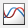Open the Simulation Data Inspector

## Syntax

``Simulink.sdi.view``

## Description

example

````Simulink.sdi.view` opens the Simulation Data Inspector. You can write a script to plot your data and customize the Simulation Data Inspector properties and then use `Simulink.sdi.view` to see the results.```

## Examples

collapse all

You can open the Simulation Data Inspector from the MATLAB® command line to visualize, inspect, and analyze your data.

`Simulink.sdi.view`

This example shows how to create a run, add data to it, and then view the data in the Simulation Data Inspector.

Create Data for the Run

Create `timeseries` objects to contain data for a sine signal and a cosine signal. Give each `timeseries` object a descriptive name.

```time = linspace(0,20,100); sine_vals = sin(2*pi/5*time); sine_ts = timeseries(sine_vals,time); sine_ts.Name = 'Sine, T=5'; cos_vals = cos(2*pi/8*time); cos_ts = timeseries(cos_vals,time); cos_ts.Name = 'Cosine, T=8';```

Create a Run and Add Data

Use the `Simulink.sdi.view` function to open the Simulation Data Inspector.

`Simulink.sdi.view`

To import data into the Simulation Data Inspector from the workspace, create a `Simulink.sdi.Run` object using the `Simulink.sdi.Run.create` function. Add information about the run to its metadata using the `Name` and `Description` properties of the `Run` object.

```sinusoidsRun = Simulink.sdi.Run.create; sinusoidsRun.Name = 'Sinusoids'; sinusoidsRun.Description = 'Sine and cosine signals with different frequencies';```

Use the `add` function to add the data you created in the workspace to the empty run.

`add(sinusoidsRun,'vars',sine_ts,cos_ts);`

Plot the Data in the Simulation Data Inspector

Use the `getSignalByIndex` function to access `Simulink.sdi.Signal` objects that contain the signal data. You can use the `Simulink.sdi.Signal` object properties to specify the line style and color for the signal and plot it in the Simulation Data Inspector. Specify the `LineColor` and `LineDashed` properties for each signal.

```sine_sig = getSignalByIndex(sinusoidsRun,1); sine_sig.LineColor = [0 0 1]; sine_sig.LineDashed = '-.'; cos_sig = sinusoidsRun.getSignalByIndex(2); cos_sig.LineColor = [0 1 0]; cos_sig.LineDashed = '--';```

Use the `Simulink.sdi.setSubPlotLayout` function to configure a `2`-by-`1` subplot layout in the Simulation Data Inspector plotting area. Then use the `plotOnSubplot` function to plot the sine signal on the top subplot and the cosine signal on the lower subplot.

```Simulink.sdi.setSubPlotLayout(2,1); plotOnSubPlot(sine_sig,1,1,true); plotOnSubPlot(cos_sig,2,1,true);```

Close the Simulation Data Inspector and Save Your Data

When you have finished inspecting the plotted signal data, you can close the Simulation Data Inspector and save the session to an MLDATX file.

`Simulink.sdi.close('sinusoids.mldatx')`

## Alternatives

You can open the Simulation Data Inspector from the Simulink® Editor toolbar with the button.

## Version History

Introduced in R2011b